Area of triangle

Chapter 9 Class 7 Perimeter and Area
Concept wise

Let us take triangle ABC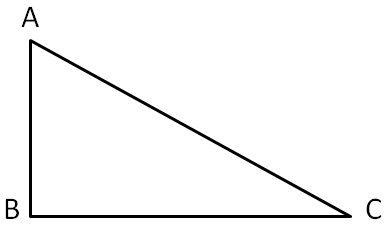Let there be another triangle which is exactly same as ∆ABC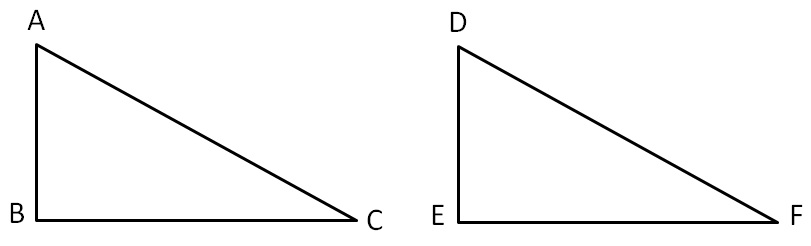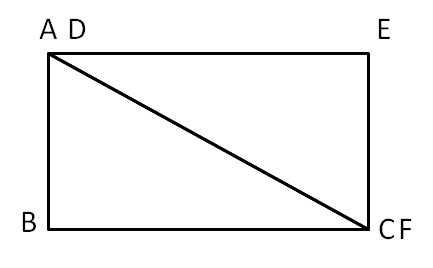If we join both these triangles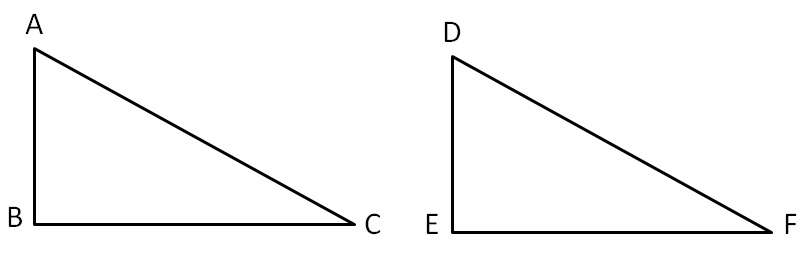We get a parallelogram ABCD

So, two equal triangles form a parallelogram

Now,

Area of parallelogram = Base × Height

Area of ∆ABC + Area of ∆DEF = b × h

Since both triangles are same,

their area will be equal

Area of ∆ABC + Area of ∆ ABC = b × h

2 Area of ∆ABC = b × h

Area of ∆ABC = b × h

Area of ∆ABC = 1/2 × b × h

So, our formula is

Area of triangle = 1/2 × b × h

#### For right angled triangle ∆ABC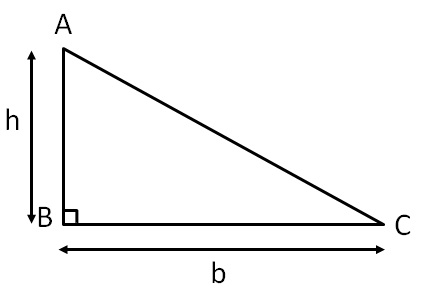Height = h = AB

Base = b = BC

So,

Area of ∆ABC = 1/2 × b × h

= 1/2 × AB × BC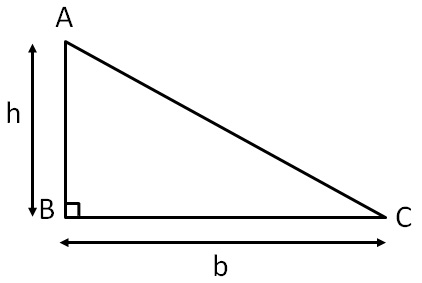Here,

base is any side of triangle

& height is perpendicular from opposite  vertex to the base

Let’s take some examples

#### Find area of Δ ABC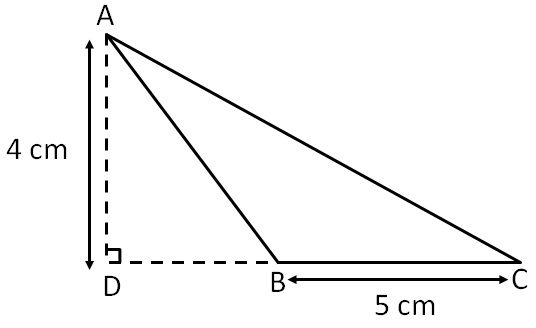In ∆ABC,

= 4 cm

Base = b = BC

= 5 cm

So,

Area of ∆ABC = 1/2 × b × h

= 1/2 × 5 × 4

= 5 × 2

= 10 cm 2

∴ Area of ∆ABC is 10 cm 2

Note: Here, height is outside the triangle

#### Find area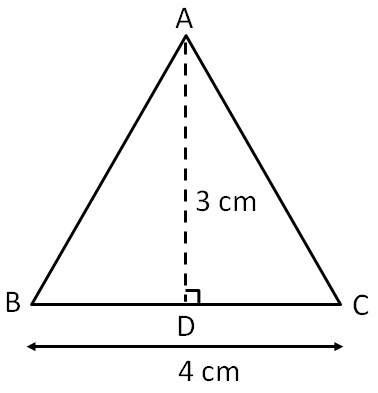In ∆ABC,

= 3 cm

Base = b = BC

= 4 cm

So,

Area of ∆ABC = 1/2 × b × h

= 1/2 × 4 × 3

= 2 × 3

= 6 cm 2

∴ Area of ∆ABC is 6 cm 2

#### Find area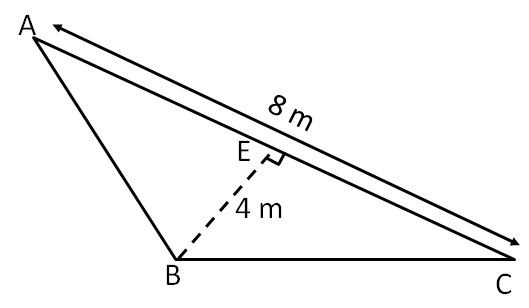In ∆ABC,

Height = h = BE

= 4 m

Base = b = AC

= 8 m

So,

Area of ∆ABC = 1/2 × b × h

= 1/2 × 8 × 4

= 4 × 4

= 16 m 2

∴ Area of ∆ABC is 16 m 2

#### Find area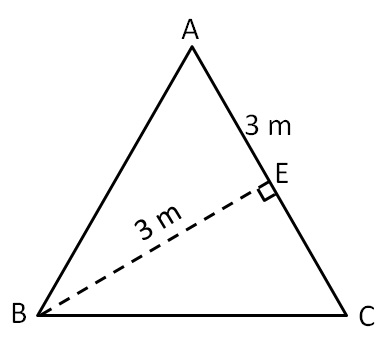In ∆ABC,

Height = h = BE

= 3 m

Base = b = AC

= 3 m

So,

Area of ∆ABC = 1/2 × b × h

= 1/2 × 3 × 3

= 1/2 × 9

= 9/2

= 4.5 m 2

∴ Area of ∆ABC is 4.5 m 2

Learn in your speed, with individual attention - Teachoo Maths 1-on-1 Class